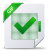Straight-line programs

Straight-line programs are a model of computation that consist in ordered lists of instructions without branching. We give a short presentation of this notion and refer to [BCS97] for more details. We will use this model of computation to describe and analyze the forthcoming recursive operators and shifted algorithms. Let R be a ring and A an R-algebra. A straight-line program (s.l.p.) is an ordered sequence of operations between elements of A. An operation of arity r is a map from a subset D of Ar to A. We usually work with the binary arithmetic operators +, −, ·: D = A2→A. We also define for r ∈ R the 0-ary operations r c whose output is the constant r and the unary scalar multiplication r × _ by r. We denote the set of all these operations by Rc and R. Let us fix a set of operations Ω, usually Ω = {+, −, ·} ∪ R ∪ Rc . An s.l.p. starts with a number ℓ of input parameters indexed from −(ℓ − 1) to 0. It has L instructions Γ1, , ΓL with Γi = (ωi ; ui,1, , ui,ri ) where −ℓ < ui,1, , ui,ri < i and ri is the arity of the operation ωi ∈ Ω. The s.l.p. Γ is executable on a= (a0, , aℓ−1) with result sequence b= (b−ℓ+1, , bL)∈Aℓ+L , if bi=aℓ−1+i whenever −(ℓ −1)6i60 and bi=ωi(bu,1, , bu,ri ) with (bu,1, , bu,ri )∈ Dωi whenever 16i6L. We say that the s.l.p. Γ computes b∈ A on the entries a1, , aℓ if Γ is executable on a1, , aℓ over A and b is a member of the result sequence. The multiplicative complexity L ∗ (Γ) of an s.l.p. Γ is the number of operations ωi that are multiplications · between elements of A. Example 2.1. Let R=Z, A=Z[X, Y ] and Γ be the s.l.p. with two input parameters indexed −1, 0 and Γ1 = (·; −1, −1), Γ2 = (·; 1, 0), Γ3 = (1c ), Γ4 = (−; 2, 3), Γ5 = (3 × _; 1). First, its multiplicative complexity is L ∗ (Γ) = 2. Then, Γ is executable on (X, Y )∈ A2 , and for this input its result sequence is (X, Y , X2 , X2 Y , 1, X2 Y −1, 3 X2 ). Remark 2.2. For the sake of simplicity, we will associate a “canonical” arithmetic expression with an s.l.p. It is the same operation as when one writes an arithmetic expression in a programming language, e.g. C, and a compiler turns it into an s.l.p. In our case, we fix an arbitrary compiler that starts by the left-hand side of an arithmetic expression. We use the binary powering algorithm to compute powers of an expression. For example, the arithmetic expression ϕ:Z  Z 4 + 1 can be represented by the s.l.p. with one argument and instructions Γ1 = (·; 0, 0), Γ2 = (·; 1, 1), Γ3 = (1c ), Γ4 = (+; 2, 3).Télécharger le document complet